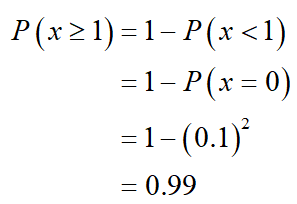# One design for a system requires the installation of two identical components. The system will work if at least one of the components works. An alternative design requires four of these components, and the system will work if at least two of the four components work. If the probability that a component works is 0.9, and if the components function independently, which design has the greater probability of functioning?

Question
1 views

One design for a system requires the installation of two identical components. The system will work if at least one of the components works. An alternative design requires four of these components, and the system will work if at least two of the four components work. If the probability that a component works is 0.9, and if the components function independently, which design has the greater probability of functioning?

check_circle

Step 1

The first design will work if at least one of the components work, the probability is,Thus, the probability of first design functioning is 0.99.

...

### Want to see the full answer?

See Solution

#### Want to see this answer and more?

Solutions are written by subject experts who are available 24/7. Questions are typically answered within 1 hour.*

See Solution
*Response times may vary by subject and question.
Tagged in